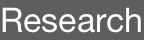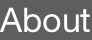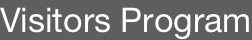Activities1. physical and mathematical relations between quantum metric and topology of chern insulators

Tomoki Ozawa

Tohoku University, Japan

31 May 2022 Tue 4 pmIBS Center for Theoretical Physics of Complex Systems (PCS), Administrative Office (B349), Theory Wing, 3rd floor

Expo-ro 55, Yuseong-gu, Daejeon, South Korea, 34126 Tel: +82-42-878-8633

Topology of two-dimensional insulators without any symmetry is characterized by Chern numbers. While Chern numbers reveal the global, topological, feature of Bloch states in the Brillouin zone, Berry curvature tells the local, geometrical, feature of Bloch states around a given momentum. Besides Berry curvature, another geometrical property called the quantum metric is naturally defined in momentum space from the structure of the Bloch states. As the name suggests, quantum metric introduces a metric structure in the tangent space of the Brillouin zone. Although Berry curvature and the quantum metric are two distinct geometrical properties, there in fact are relations between the two. From these relations, one can derive an inequality between the Chern number and the area of the Brillouin zone measured with respect to the quantum metric. We study various aspects of this inequality both from physical and mathematical perspectives. We find that we can infer topological properties just by examining properties of the quantum metric in many cases. In particular, when models approach the Landau level limit in an appropriate sense, the inequality tends to saturate and approach an equality. On a more mathematical side, the condition of the inequality becoming equality is found be related to the holomorphic nature of the Bloch states. We find that, when the equality holds, momentum space inherits the Kähler manifold structure from the complex projective space, which is a space of states in which Bloch states live. We also propose a way to construct a geometrically uniform Chern insulators obeying the equality, using holomorphic functions defined in momentum space.  Such a geometrically uniform Chern insulators with holomorphic structures are known to be useful in obtaining fractional Chern insulators using analogy from the fractional quantum Hall states.